# Equations of Motion for a Particle (Part - 1) Civil Engineering (CE) Notes | EduRev

## Civil Engineering (CE) : Equations of Motion for a Particle (Part - 1) Civil Engineering (CE) Notes | EduRev

The document Equations of Motion for a Particle (Part - 1) Civil Engineering (CE) Notes | EduRev is a part of the Civil Engineering (CE) Course Introduction to Dynamics and Vibrations- Notes, Videos, MCQs.
All you need of Civil Engineering (CE) at this link: Civil Engineering (CE)

Analyzing motion of systems of particles

In this chapter, we shall discuss

1. The concept of a particle
2. Position/velocity/acceleration relations for a particle
3. Newton’s laws of motion for a particle
4. How to use Newton’s laws to calculate the forces needed to make a particle move in a particular way
5. How to use Newton’s laws to derive `equations of motion’ for a system of particles
6. How to solve equations of motion for particles by hand or using a computer.

The focus of this chapter is on setting up and solving equations of motion – we will not discuss in detail the behavior of the various examples that are solved.

3.1 Equations of motion for a particle

3.1 Equations of motion for a particle We start with some basic definitions and physical laws

3.1.1 Definition of a particle

A `Particle’ is a point mass at some position in space. It can move about, but has no characteristic orientation or rotational inertia. It is characterized by its mass. Examples of applications where you might choose to idealize part of a system as a particle include:

1. Calculating the orbit of a satellite – for this application, you don’t need to know the orientation of the satellite, and you know that the satellite is very small compared with the dimensions of its orbit.

2. A molecular dynamic simulation, where you wish to calculate the motion of individual atoms in a material. Most of the mass of an atom is usually concentrated in a very small region (the nucleus) in comparison to inter-atomic spacing. It has negligible rotational inertia. This approach is also sometimes used to model entire molecules, but rotational inertia can be important in this case.

Obviously, if you choose to idealize an object as a particle, you will only be able to calculate its position. Its orientation or rotation cannot be computed.

3.1.2 Position, velocity, acceleration relations for a particle (Cartesian coordinates)

In most practical applications we are interested in the position or the velocity (or speed) of the particle as a function of time. But Newton’s laws will only tell us its acceleration. We therefore need equations that relate the position, velocity and acceleration.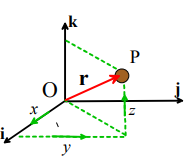Position vector: In most of the problems we solve in this course, we will specify the position of a particle using the Cartesian components of its position vector with respect to a convenient origin. This means

1. We choose three, mutually perpendicular, fixed directions in space. The three directions are described by unit vectors {i,j,k}

2. We choose a convenient point to use as origin

3. The position vector (relative to the origin) is then specified by the three distances (x,y,z) shown in the figure.

r = x(t)i + y(t) j + z(t)k

In dynamics problems, all three components can be functions of time.

Velocity vector: By definition, the velocity is the derivative of the position vector with respect to time (following the usual machinery of calculus)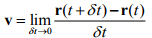Velocity is a vector, and can therefore be expressed in terms of its Cartesian components

v = vxi + vyj + vzk

You can visualize a velocity vector as follows

• The direction of the vector is parallel to the direction of motion
• The magnitude of the vector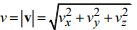is the speed of the particle (in meters/sec, for example).

When both position and velocity vectors are expressed in terms Cartesian components, it is simple to calculate the velocity from the position vector. For this case, the basis vectors {i,j,k} are constant (independent of time) and so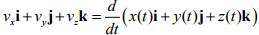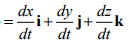This is really three equations – one for each velocity component, i.e.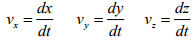Acceleration vector: The acceleration is the derivative of the velocity vector with respect to time; or, equivalently, the second derivative of the position vector with respect to time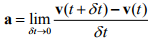The acceleration is a vector, with Cartesian representation a = axi + ayj + azk .

Like velocity, acceleration has magnitude and direction. Sometimes it may be possible to visualize an acceleration vector – for example, if you know your particle is moving in a straight line, the acceleration vector must be parallel to the direction of motion; or if the particle moves around a circle at constant speed, its acceleration is towards the center of the circle. But sometimes you can’t trust your intuition regarding the magnitude and direction of acceleration, and it can be best to simply work through the math.

The relations between Cartesian components of position, velocity and acceleration are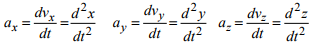3.1.3 Examples using position-velocity-acceleration relations

It is important for you to be comfortable with calculating velocity and acceleration from the position vector of a particle. You will need to do this in nearly every problem we solve. In this section we provide a few examples. Each example gives a set of formulas that will be useful in practical applications.

Example 1: Constant acceleration along a straight line. There are many examples where an object moves along a straight line, with constant acceleration. Examples include free fall near the surface of a planet (without air resistance), the initial stages of the acceleration of a car, or and aircraft during takeoff roll, or a spacecraft during blastoff.

Suppose that

The particle moves parallel to a unit vector i
The particle has constant acceleration, with magnitude a
At time t = t0 the particle has speed v0
At time t = t0 the particle has position vector r = x0i

The position, velocity acceleration vectors are then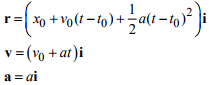Verify for yourself that the position, velocity and acceleration (i) have the correct values at t=0 and (ii) are related by the correct expressions (i.e. differentiate the position and show that you get the correct expression for the velocity, and differentiate the velocity to show that you get the correct expression for the acceleration).

HEALTH WARNING: These results can only be used if the acceleration is constant. In many problems acceleration is a function of time, or position – in this case these formulas cannot be used. People who have taken high school physics classes have used these formulas to solve so many problems that they automatically apply them to everything – this works for high school problems but not always in real life!

Example 2: Simple Harmonic Motion: The vibration of a very simple springmass system is an example of simple harmonic motion.

In simple harmonic motion (i) the particle moves along a straight line; and (ii) the position, velocity and acceleration are all trigonometric functions of time.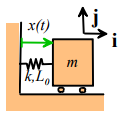For example, the position vector of the mass might be given by

r = x(t)i = (X0 + ΔX sin(2πt/ T)) i

Here X0 is the average length of the spring, X0 +ΔX is the maximum length of the spring, and T is the time for the mass to complete one complete cycle of oscillation (this is called the `period of oscillation).

Harmonic vibrations are also often characterized by the frequency of vibration:

• The frequency in cycles per second (or Hertz) is related to the period by f=1/T
• The angular frequency is related to the period by ω = 2π / T

The motion is plotted in the figure on the right

The velocity and acceleration can be calculated by differentiating the position, as follows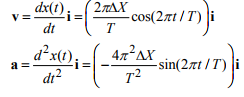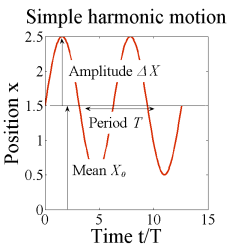Note that:

• The velocity and acceleration are also harmonic, and have the same period and frequency as the displacement.
• If you know the frequency, and amplitude and of either the displacement, velocity, or acceleration, you can immediately calculate the amplitudes of the other two. For example, if ΔX , ΔV , ΔA denote the amplitudes of the displacement, velocity and acceleration, we have that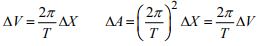Example 3: Motion at constant speed around a circular path Circular motion is also very common – examples include any rotating machinery, vehicles traveling around a circular path, and so on.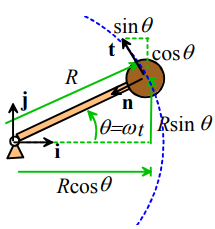• The angle θ increases at constant rate.  We can write θ =ωt , where ω is the (constant) angular speed of the shaft, in radians/seconds.
• The speed of the particle is related to ω by V =Rω. To see this, notice that the circumferential distance traveled by the particle is s = Rθ .  Therefore, V = ds / dt = Rdθ/ dt = Rω.

For this example the position vector is

r = R cosθ i+R sinθ j

The velocity can be calculated by differentiating the position vector.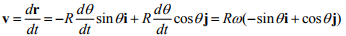Here, we have used the chain rule of differentiation, and noted that dθ / dt = ω .

The acceleration vector follows as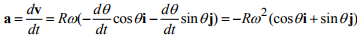Note that

(i) The magnitude of the velocity is V = Rω, and its direction is (obviously!) tangent to the path (to see this, visualize (using trig) the direction of the unit vector t = (-sinθi+cosθj)

(ii) The magnitude of the acceleration is Rω2 and its direction is towards the center of the circle. To see this, visualize (using trig) the direction of the unit vector n = (-cosθi + sinθj)

We can write these mathematically as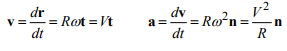Example 4: More general motion around a circular path

We next look at more general circular motion, where the particle still moves around a circular path, but does not move at constant speed. The angle θ is now a general function of time.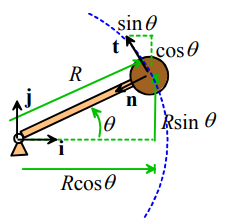We can write down some useful scalar relations:

• Angular rate: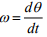• Angular acceleration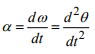• Speed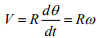• Rate of change of speed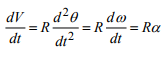We can now calculate vector velocities and accelerations

r = R cosθ i+R sinθ j

The velocity can be calculated by differentiating the position vector.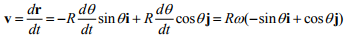The acceleration vector follows as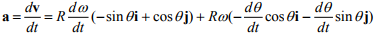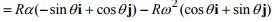It is often more convenient to re-write these in terms of the unit vectors n and t normal and tangent to the circular path, noting that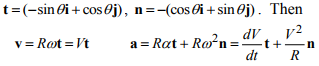These are the famous circular motion formulas that you might have seen in physics class.

Using MAPLE to differentiate position-velocity-acceleration relations

If you find that your calculus is a bit rusty you can use MAPLE to do the tedious work for you. You already know how to differentiate and integrate in MAPLE – the only thing you may not know is how to tell MAPLE that a variable is a function of time. Here’s how this works. To differentiate the vector

r = x(t)i + y(t) j + z(t)k

you would type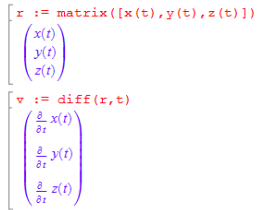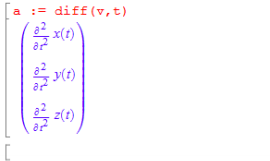It is essential to type in the (t) after x,y,and z – if you don’t do this, Mupad assumes that these variables are constants, and takes their derivative to be zero. You must enter (t) after _any_ variable that changes with time.

Here’s how you would do the circular motion calculation if you only know that the angle θ is some arbitrary function of time, but don’t know what the function is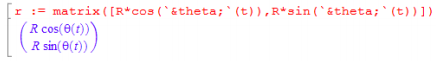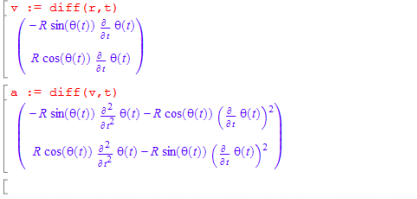As you’ve already seen in EN3, Matlab can make very long and complicated calculations fairly painless. It is a godsend to engineers, who generally find that every real-world problem they need to solve is long and complicated. But of course it’s important to know what the program is doing – so keep taking those math classes…

Offer running on EduRev: Apply code STAYHOME200 to get INR 200 off on our premium plan EduRev Infinity!

## Introduction to Dynamics and Vibrations- Notes, Videos, MCQs

20 videos|53 docs

,

,

,

,

,

,

,

,

,

,

,

,

,

,

,

,

,

,

,

,

,

;